# What is a proportion?

A proportion is really two ratios that are equivalent to each other. Here is an example:There are several ways to tell if two ratios form a proportion.

1st method: Check to see if the same scale factor was used on top and bottom.2nd method: Try and simplify one or both of the ratios.

In this example, we could reduce the second ratio. Check it out:Once again, we can see that the two ratios are equivalent. Therefore, they must form a proportion.

3rd method: Cross products: Multiply the numbers that are diagonal to each other. If the products are equal, the two ratios form a proportion.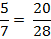5 x 28 = 140

7 x 20 = 140

Check out what happens when you do not have a proportion: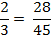2 x 45 = 90

3 x 28 = 84

Here, the cross products are not the same. This shows that the two ratios are not a proportion.

These steps are also useful in finding the missing number in a proportion.

Examples: Here are some examples of finding the missing number in a proportion using all three methods.

1.)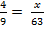2.)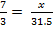3.)This is a good example to use the simplifying method. We can see that the first ratio can be simplified because both 30 and 45 have a common factor. However, you may not want to simplify to lowest terms. Instead, we can simplify both top and bottom by dividing by 3 so that the numerators will match.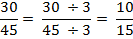Here we can see that x must be 15.

Depending on the type of ratio you might be working with, one method may be easier to use than another. If you cannot see anything to reduce or cannot come up with a scale factor, the cross products method always work.

Math
What is a Ratio?
Equivalent Ratios
Unit Rates
Ratios
Fractions
Factors